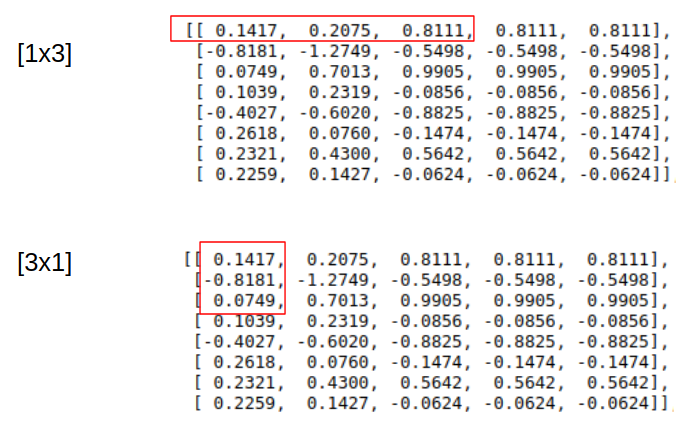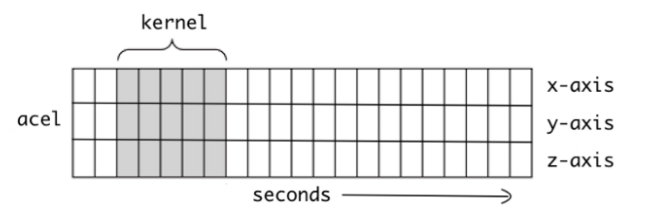# Dilated causal convolutions

Hi,

I want to replicate this dilated causal convolution:

m being some different categories, k being time steps and 4 the channels.

I defined the convolutional layer like this:
`nn.Conv1d(in_channels=4, out_channels=8, kernel_size=(1,3), stride=1, padding=2, dilation=1)`

given m=3 and k=5 it gives me the following error:

RuntimeError: Expected 4-dimensional input for 4-dimensional weight [8, 4, 1, 3], but got 3-dimensional input of size [3, 4, 5] instead

If I change the Kernel size to 1 I get an output with the wrong shape:
torch.Size([3, 8, 9])

and if I set the kernel to 3 the output as the shape:
torch.Size([3, 8, 7])

But it has never the output shape [3, 5, 8.]
Am I missing something here?

IIRC causal convolutions use one-sided paddings. And kernel size should be 1d (3) for conv1d.

Can you help me with this as well?

for padding same i would do
` left = (kernel_size - 1) // 2`
` right = (kernel_size - 1) - left`
`F.pad(x, (left,right)`
however im unsure about the kernel size [m x 1] again . Would it for my example just be 3?

Yes, 3x1 is to do the same using conv2d

As for padding, I think it should work like:

``````abcd
ABCDEF      //conv output
ABCD         //causal shift
``````

So you either cut EF or don’t use `",,"` padding tail.

Let me try to repeat this.
So you say for the correlational layer with padding “same”:

correl = nn.Conv2d(in_channels=8, out_channels=8, kernel_size=(3,1), stride=1)

But I really don’t understand why they write [3x1] in the paper. But then I’m supposed to use a kernel_size of 3 which is a 3x3 matrix?

in pytorch conv1d dispatches to conv2d, adding a fake dimension, I guess in their framework something similar happens, or they have other reasons to unsqueeze input to 4d.

I didn’t say to use 3 with conv2d

in pytorch conv1d dispatches to conv2d, adding a fake dimension, I guess in their framework something similar happens, or they have other reasons to unsqueeze input to 4d.

Oh I understand.

I didn’t say to use 3 with conv2d

Sry if I did understand you wrong. But what did you mean?

As I understand it the [1x3] or kernel size 3 for conv1d with dilation learns the causality of each channel. However, [3x1] learns the correlation between these channels.But I’m unsure how to express that [3x1] filter with conv1d.

Kernel size is not about channels, it is a “window” size in spatial/time dimension, 1d window for 3d input, 2d for 4d input. Tensor format is (batch, channels, time), with kernel_size 3 you’re aggregating 3 timesteps, while in_channels -> out_channels map is dense.

yes thats what I tried to visualize with the [1x3] red rectangle. a window of three time steps in the time dimension.
But what im trying to understand is the [3x1] window and how this is expressed with pytorch conv1d.

For the first picture, you’d normally get 8 channels x 3 timesteps windows, unless you use channel groups (groups=8 parameter would divide channels as 8/8).

There is no built-in support for uneven channel groups (e.g. 3/8 channels). You can implement that with masks (F.conv1d(input, weight*mask, …)), but it is a bit cumbersome.

@googlebot thanks for you help I think I understood it now…

so for the conv1d with kernel size 3 it is like thisthe filter with size 5 moving over the time steps. and depending on how “high” the next matrix should be the more filters we use. so for 8 output channels 8 filters etc.

now I’m thinking of the correlation convolution. just imagine there would be 3 acel matrices of three different objects… like in the image below. with a [mx1] kernel I want to go over each input channel of the three elements. I would pad one matrix m0 and one m4 so that I would be able to get 3 output matrices.

so as in the picture. input would be (3,3,5) and a possible output (3,3,5). by that having 3 filters. I’m just unsure if this would be possible.

I was also considering to just “.transpose(0,2)” the matrix. so that the batch_size becomes the time steps and the time steps the batch size. and then use a regular conv1d with kernel size m the previously batch size and now time steps.

Hope I could make it somehow understandable. Do you think this would be possible without transposing?

Is your object set intrinsically ordered or should output be permutation invariant? Is it a variable size set?

variable size is set, yes.

yes, there is an order in terms of different channel features and somehow they are depending on each other.

Then perhaps 4d input is appropriate:
(batch_size=1, channels=3 [xyz], objects=m, timesteps=k)

note that batch_size=m is initially incorrect if objects are not independent (unless later layers add autoregressive dependencies)

With above 4d input, you can perhaps do two separate causal convolutions 1x3 and 3x1. That’s not something I’ve ever done, so not sure.